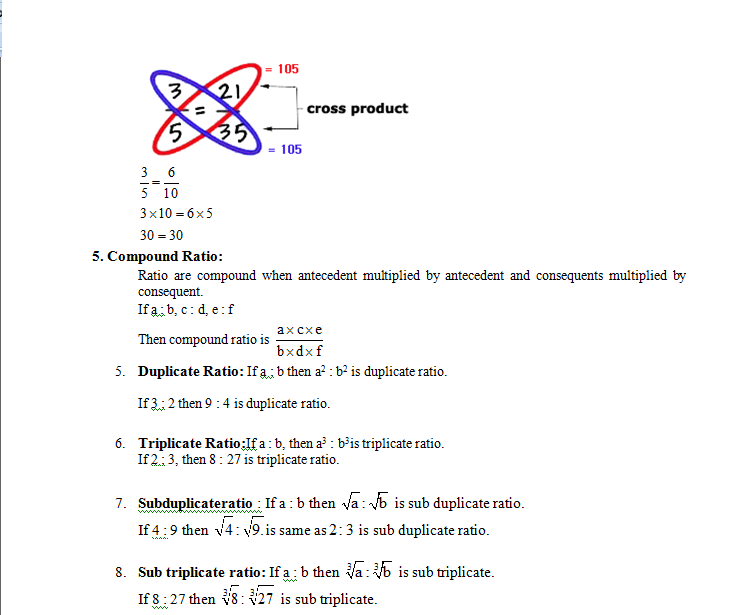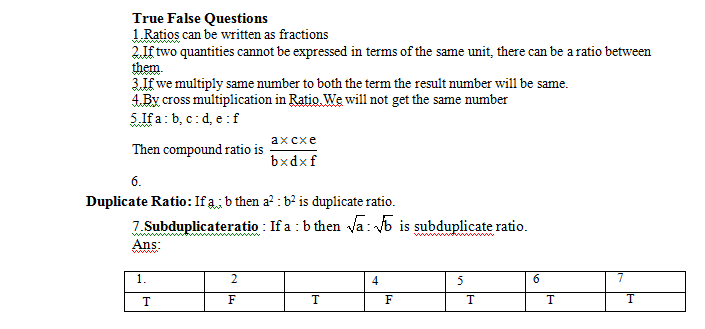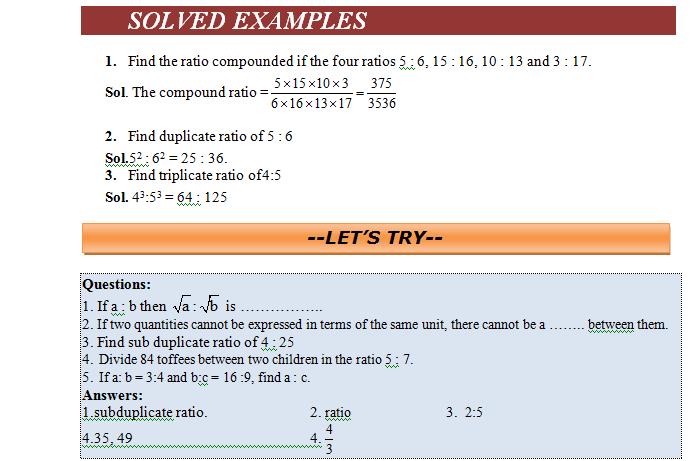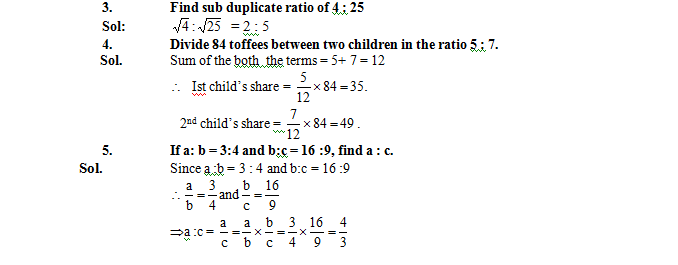# Introduction

## Comparing Quantities

A ratio is a comparison of two similar quantities obtained by dividing one quantity by the other. Ratios are written with the : symbol.

We can understand ratio in different form as

Since a ratio is only a comparison or relation between quantities, it is an abstract number.

For instance we consider the ratio of 10 km to 5 km is only 2, not 2 km.Because it is unit less.

Ex:The ratio of 10 to 5 is

10 ÷ 5 = 10/5 we can write this as

10: 5 = 2

• we have to write down only the number not unit of the quantities.Because it is unit less.
• Ratios can be written as fractions. They also have all the properties of fractions that you have already learned in the previous part of this chapter.
• The ratio of 10 to 5 should be stated as 2 to 1, but common usage has shortened the expression of ratios to be called simply 2.
• If two quantities cannot be expressed in terms of the same unit, there cannot be a ratio between

them. E.g.A man has to travel 50 k.m. and time taken is 2 hours. In this case we will not take the ratio of these quantity. Because we know that the distance covered divided by time is velocity this is not ration quantity.

The number of times one quantity contains another quantity of same kind is called the ‘RATIO’ of the two quantities. Clearly, the ratio of two quantities is equivalent to the fraction that one quantity is of the other.

### Properties of Ratio

• If we multiply same number to both the term the result number will be same. Same as we have already studied in fraction.
• Similarly Ratio is not altered by dividing both its terms by the same number.
• If we add or subtract same number with both the term then the result will alter or our result will change.
• By cross multiplication in Ratio.We will get same numberTo score More in your class 7 refer NCERT solutions for class 7 Maths